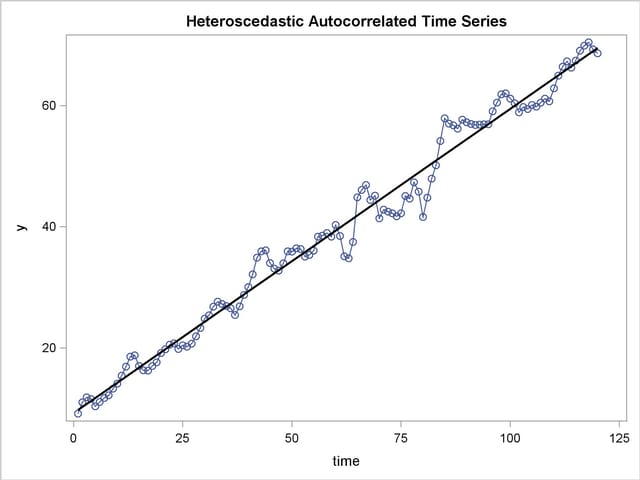The AUTOREG Procedure

One of the key assumptions of the ordinary regression model is that the errors have the same variance throughout the sample. This is also called the homoscedasticity model. If the error variance is not constant, the data are said to be heteroscedastic.

Since ordinary least squares regression assumes constant error variance, heteroscedasticity causes the OLS estimates to be inefficient. Models that take into account the changing variance can make more efficient use of the data. Also, heteroscedasticity can make the OLS forecast error variance inaccurate since the predicted forecast variance is based on the average variance instead of the variability at the end of the series.

To illustrate heteroscedastic time series, the following statements re-create the simulated series Y. The variable Y has an error variance that changes from 1 to 4 in the middle part of the series. The length of the series is also extended 120 observations.

```   data a;
ul = 0; ull = 0;
do time = -10 to 120;
s = 1 + (time >= 60 & time < 90);
u = + 1.3 * ul - .5 * ull + s*rannor(12346);
y = 10 + .5 * time + u;
if time > 0 then output;
ull = ul; ul = u;
end;
run;

title 'Heteroscedastic Autocorrelated Time Series';
proc sgplot data=a noautolegend;
series x=time y=y / markers;
reg x=time y=y / lineattrs=(color=black);
run;
```

The simulated series is plotted in Figure 8.10.

Figure 8.10 Heteroscedastic and Autocorrelated SeriesTo test for heteroscedasticity with PROC AUTOREG, specify the ARCHTEST option. The following statements regress Y on TIME and use the ARCHTEST option to test for heteroscedastic OLS residuals. The DWPROB option is also used to test for autocorrelation.

```   /*-- test for heteroscedastic OLS residuals --*/
proc autoreg data=a;
model y = time / nlag=2 archtest dwprob;
output out=r r=yresid;
run;
```

The PROC AUTOREG output is shown in Figure 8.11. The Q statistics test for changes in variance across time by using lag windows ranging from 1 through 12. (See the section Heteroscedasticity and Normality Tests for details.) The p-values for the test statistics are given in parentheses. These tests strongly indicate heteroscedasticity, with p < 0.0001 for all lag windows.

The Lagrange multiplier (LM) tests also indicate heteroscedasticity. These tests can also help determine the order of the ARCH model appropriate for modeling the heteroscedasticity, assuming that the changing variance follows an autoregressive conditional heteroscedasticity model.

Figure 8.11 Heteroscedasticity Tests
 Heteroscedastic Autocorrelated Time Series

The AUTOREG Procedure

Dependent Variable y

 Heteroscedastic Autocorrelated Time Series

The AUTOREG Procedure

Ordinary Least Squares Estimates
SSE 690.266009 DFE 118
MSE 5.84971 Root MSE 2.41862
SBC 560.070468 AIC 554.495484
MAE 3.72760089 AICC 554.598048
MAPE 5.24916664 Regress R-Square 0.9814
Durbin-Watson 0.4060 Total R-Square 0.9814

Q and LM Tests for ARCH Disturbances
Order Q Pr > Q LM Pr > LM
1 37.5445 <.0001 37.0072 <.0001
2 40.4245 <.0001 40.9189 <.0001
3 41.0753 <.0001 42.5032 <.0001
4 43.6893 <.0001 43.3822 <.0001
5 55.3846 <.0001 48.2511 <.0001
6 60.6617 <.0001 49.7799 <.0001
7 62.9655 <.0001 52.0126 <.0001
8 63.7202 <.0001 52.7083 <.0001
9 64.2329 <.0001 53.2393 <.0001
10 66.2778 <.0001 53.2407 <.0001
11 68.1923 <.0001 53.5924 <.0001
12 69.3725 <.0001 53.7559 <.0001

Variable DF Estimate Standard Error t Value Approx
Pr > |t|
Intercept 1 9.2217 0.4444 20.75 <.0001
time 1 0.5024 0.006374 78.83 <.0001Previous Page | Next Page | Top of Page